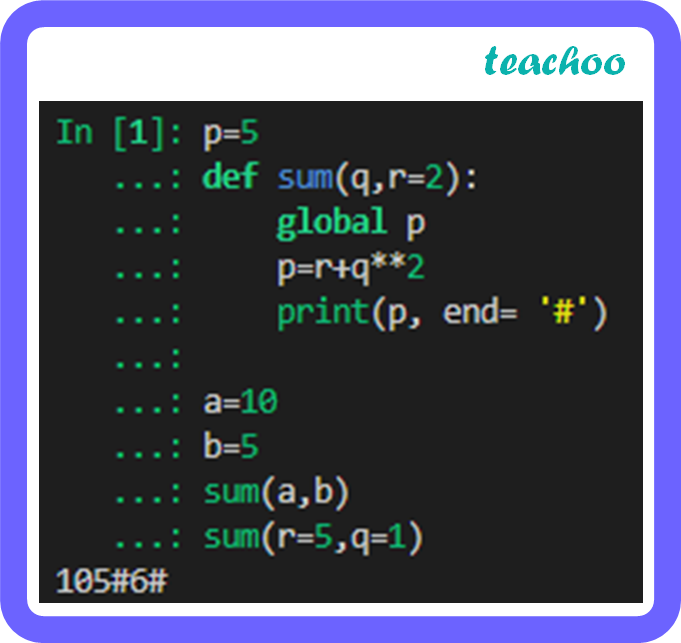CBSE Class 12 Sample Paper for 2023 Boards

Computer Science - Class 12
Solutions to CBSE Sample Paper - Computer Science Class 12

## (a) Write the output of the code given below:

``` p=5 ```

``` def sum(q,r=2): ```

``` global p ```

``` p=r+q**2 ```

``` print(p, end= '#') ```

``` a=10 ```

``` b=5 ```

``` sum(a,b) ```

``` sum(r=5,q=1) ```Output: 105#6#

Explanation:

In the first function call, a and b are passed as parameters. This assigns ‘q’ the value 10 and ‘r’ the value 5. This gives p the value 105 and it is printed with a ‘#’ after.

In the second function call, ‘q’ is assigned the value 1 and ‘r’ is assigned the value 5. This gives p the value 6.

Now the output will be: 105#6#

Learn in your speed, with individual attention - Teachoo Maths 1-on-1 Class